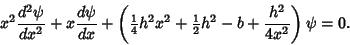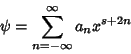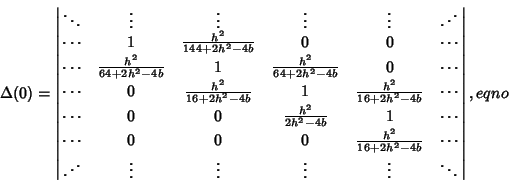## Hill Determinant

A Determinant which arises in the solution of the second-order Ordinary Differential Equation(1)

Writing the solution as a Power Series(2)

gives a Recurrence Relation(3)

The value ofcan be computed using the Hill determinant(4)

where(5)(6)(7)

andis the variable to solve for. The determinant can be given explicitly by the amazing formula(8)

where(9)

leading to the implicit equation for,(10)